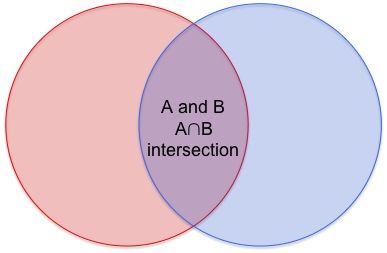September 29, 2012

Math provides a way to visualize and comprehend concepts from disciplines far and wide. As such, appropriate use of language is crucial in expressing ideas from these disciplines, including mathematics. Unfortunately, students often do not see the connection between mathematics and reading/writing.

An important example is the use of conjunctions such as “and” and “or.” In a Venn Diagram, the probability of A and B is the intersection, and the probability of A or B is the union.Prepositions such as “to” and “from” are also important to use correctly, but tricky as well. For example, if the mean and standard deviation of a normal distribution are 50 and 10, respectively, then both 40 and 60 are one standard deviation from the mean.

Besides using words, students must use mathematical language appropriately to convey ideas. One example we looked at during BAMP’s first Saturday Seminar was:

Scott gave 1/3rd of his marbles to Rebecca, then gave John 1/4th of the remaining marbles. After giving these marbles to Rebecca and John, Scott was left with 24 marbles.

Before we start asking students questions – solve this, solve that – how about students first use math to visualize the situation and verbalize this visual. Use symbols to describe the situation and use language to explain the symbols.Too often, math problems jump straight from the information to the question. This causes students to immediately focus on the question; it’s not in students’ nature to take the necessary time to first digest the information. By first presenting the information, a math “problem” becomes an “exercise.”

A group of researchers at Stanford University study language, literacy, and learning in math and science. They show how language goes hand-in-hand with learning, understanding, and articulating math.

Mathematics, the Common Core, and Language: Recommendations for Mathematics Instruction for ELs Aligned with the Common Core
By Judit Moschkovich, University of California, Santa Cruz

Writing and speaking are fundamental components of mathematical science. Even those in STEM careers must be able to clearly articulate their thoughts. One of the teachers at today’s BAMP Saturday Seminar told me that her daughter, an English major, was hired by Facebook to help the software engineers and computer scientists report their work. In class and at home, it is important that students constantly strive to communicate mathematical ideas not only in symbols, diagrams, and graphs, but in words.